# PostgreSQL Tutorial: DENSE_RANK Function

August 7, 2023

Summary: in this tutorial, you are going to learn how to use the PostgreSQL `DENSE_RANK()` function to assign a rank to each row within a partition of a result set, with no gaps in ranking values.

## Introduction to PostgreSQL `DENSE_RANK()` function

The `DENSE_RANK()` assigns a rank to every row in each partition of a result set. Different from the `RANK()` function, the `DENSE_RANK()` function always returns consecutive rank values.

For each partition, the `DENSE_RANK()` function returns the same rank for the rows which have the same values

The following shows the syntax of the `DENSE_RANK()` function:

``````DENSE_RANK() OVER (
[PARTITION BY partition_expression, ... ]
ORDER BY sort_expression [ASC | DESC], ...
)
``````

The `DENSE_RANK()` function is applied to every row in each partition defined by the `PARTITION BY` clause, in the sort order specified by `ORDER BY` clause. It will reset the rank when crossing the partition boundary.

The `PARITION BY` clause is optional. If you skip it, the `DENSE_RANK()` function will treat the whole result set as a single partition.

## PostgreSQL `DENSE_RANK()` function demo

First, create a table named `dense_ranks` that has one column:

``````CREATE TABLE dense_ranks (
c VARCHAR(10)
);
``````

Second, insert some rows into the `dense_ranks` table:

``````INSERT INTO dense_ranks(c)
VALUES('A'),('A'),('B'),('C'),('C'),('D'),('E');
``````

Third, query data from the `dense_ranks` table:

``````SELECT c from dense_ranks;
``````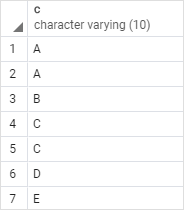Fourth, use the `DENSE_RANK()` function to assign a rank to each row in the result set:

``````SELECT
c,
DENSE_RANK() OVER (
ORDER BY c
) dense_rank_number
FROM
dense_ranks;
``````

Here is the output: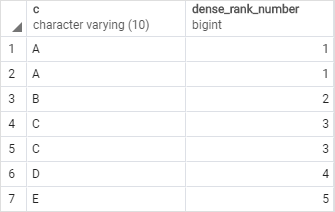## PostgreSQL `DENSE_RANK()` function examples

We will use the `products` table to demonstrate the `DENSE_RANK()` function.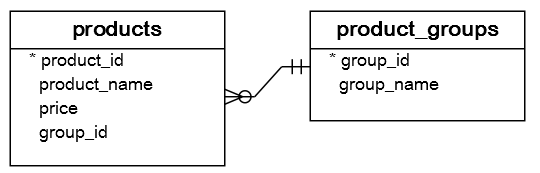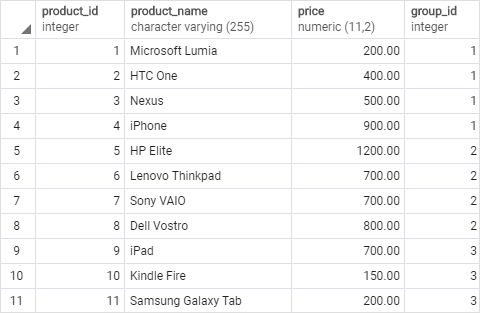### 1) Using PostgreSQL `DENSE_RANK()` function over a result set example

This statement uses the `DENSE_RANK()` function to rank products by list prices:

``````SELECT
product_id,
product_name,
price,
DENSE_RANK () OVER (
ORDER BY price DESC
) price_rank
FROM
products;
``````

Here is the output: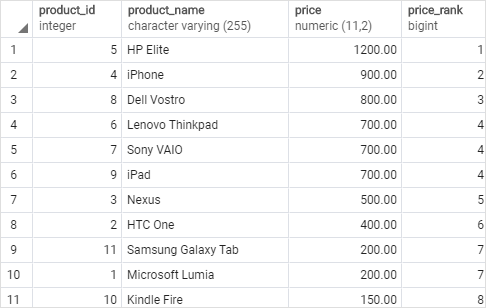In this example, we skipped the `PARTITION BY` clause, therefore, the `DENSE_RANK()` function treated the whole result set as a single partition.

The `DENSE_RANK()` function assigned a rank to each product based on the price order from high to low specified by the `ORDER BY` clause.

### 2) Using PostgreSQL `DENSE_RANK()` function over partitions example

The following example assigns a rank to every product in each product group:

``````SELECT
product_id,
product_name,
group_id,
price,
DENSE_RANK () OVER (
PARTITION BY group_id
ORDER BY price DESC
) price_rank
FROM
products;
``````

This picture shows the output: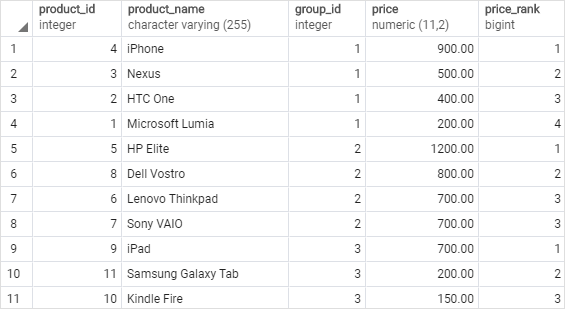In this example, the `PARTITION BY` clause distributed the products into product groups. The `ORDER BY` clause sorted products in each group by their prices from high to low to which the `DENSE_RANK()` function is applied.

### 3) Using PostgreSQL `DENSE_RANK()` function with a CTE example

The following statement uses the `DENSE_RANK()` function with a CTE to return the most expensive product in each product group:

``````WITH cte AS(
SELECT
product_id,
product_name,
group_id,
price,
DENSE_RANK () OVER (
PARTITION BY group_id
ORDER BY price DESC
) price_rank
FROM
products
)
SELECT
product_id,
product_name,
price
FROM
cte
WHERE
price_rank = 1;
``````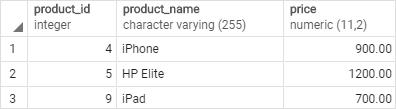In this tutorial, you have learned how to use the PostgreSQL `DENSE_RANK()` function to calculate a rank to each row within a partition of a result set, with no gaps in rank values.